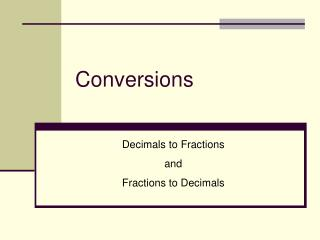DownloadDownload PresentationConversions

# Conversions

Télécharger la présentation## Conversions

- - - - - - - - - - - - - - - - - - - - - - - - - - - E N D - - - - - - - - - - - - - - - - - - - - - - - - - - -
##### Presentation Transcript

1. Conversions Decimals to Fractions and Fractions to Decimals

2. What are we converting today? Today you will convert decimals to fractions and fractions to decimals

3. Why is this important? • There are different ways to write the same number.  One number can be expressed in different ways.

4. How to Convert Decimals to Fractions Use the place value of the last digit in the number to determine what the denominator of the fraction will be.

5. How to Convert Decimals to Fractions .5 The 5 is in the tenths place 5 10

6. How to Convert Decimals to Fractions .84 The 4 is in the hundredths place 84 100

7. What if there is a whole number before the decimal point? 1.59 The 9 is in the hundredths place. The whole number goes in front of the fraction. 59 1 100

8. What if there is a whole number before the decimal point? 25.5 The 5 is in the tenths place. The whole number goes in front of the fraction. 5 25 10

9. How to Convert Fractions to Decimals 1. Read the fraction2. Place the last digit of the numerator in the place named.3. Fill in the remaining digits 23 This is the hundredths place so the 3 needs to be in the hundredths place. 100 3 2 .23

10. How to Convert Fractions to Decimals 7 This is the tenths place so the 7 needs to be in thetenths place. 10 7 .7

11. How to Convert Fractions to Decimals 4 This is the hundredths place so the 4 needs to be in thehundredths place. 100 4 0 .04

12. How to Convert Fractions to Decimals 2 This is the tenths place so the 2 needs to be in the tenths place. 10 2 .2

13. What if there is a whole number before the fraction? 56 This is the hundredths place so the 7 needs to be in thehundredths place. Now place the whole number infront. 3 100 6 3 5 3.56

14. How to Convert Fractions to Decimals 3 This is the tenths place so the 4 needs to be in thetenths place. 24 10 24.3

15. Now you try